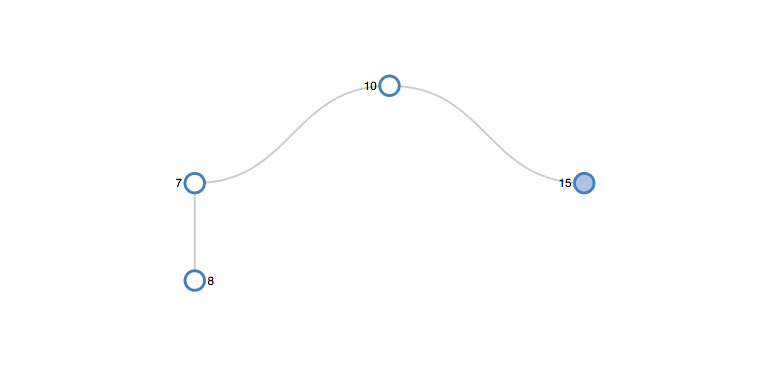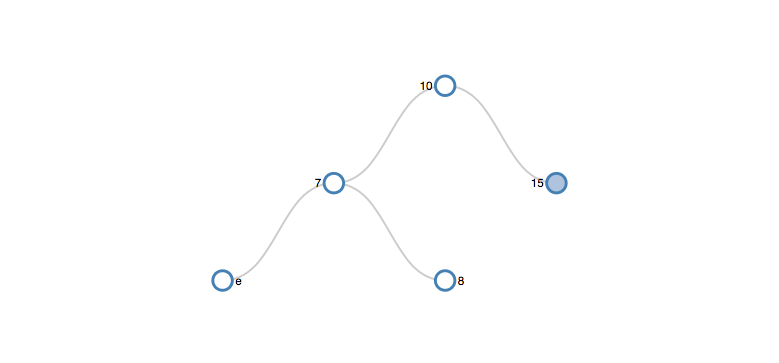Blog Portfolio

# Binary Search Tree

###### Sat, Apr 8, 2017

This post will discuss how to connect a BST implementation to d3.js.

I recently implemented a Binary Search Tree in JavaScript. It was a fun exercise and a great opportunity to use ES6 Classes.

Below is my code for the Binary Search Tree class, Node class, and their insertion methods.

```/* Binary Search Tree Class */
class BinarySearchTree {
// constructor
constructor() {
this.root = null;
}

// insert
insert(value) {
if (this.root == null) {
this.root = new Node(value);
return true;
} else {
return this.root.insert(new Node(value));
}
}
}

/* Node Class */
class Node {
constructor(value) {
this.value = value;
this.left = null;
this.right = null;
this.parent = null;
}
// insert
insert(node) {
if (node.value == this.value) {
return false;
// check left subtree
} else if (node.value < this.value) {
if (this.left != null) {
return this.left.insert(node);
} else {
this.left = node;
node.parent = this;
return true;
}
// check right subtree
} else {
if (this.right != null) {
return this.right.insert(node);
} else {
this.right = node;
node.parent = this;
return true;
}
}
}
}
```

After I finished my implementation I decided to use d3.js to visualize my BST.

To start I adapted d3noob’s block to make a program that visualized a vertical tree from a hardcoded dataset.

See the Pen D3.js Vertical Tree by Alex Katz (@katzkode) on CodePen.

In order to visualize a tree with d3.js, the following occurs:

1. The SVG is configured.
2. A d3 tree layout is initialized
3. A call is made to d3.hierarchy
4. The nodes are drawn

Even though there are a number of steps, only one part of the code needs to be altered to visualize an instance of my BST. This is the the call to `d3.hierarchy`.

```root = d3.hierarchy(treeData, function(d){
return d.children;
});
```

The function `d3.hierarchy(data, children)` takes in two parameters.

1. An object that represents the root node of a dataset
2. A children accessor function

d3.hierarchy starts with the root and invokes the accessor function for each node. The accessor function must return an array of children or null if there are no children. The hierarchy function gives each node the properties: data, depth, height, parent, children, and value.

`hierarchy` returns the root node.

The first part of the hierarchy call that needs to be changed is the data. Instead of passing the original hardcoded data set, pass a BST instance’s root node.

```// Assigns parent, children, height, depth
root = d3.hierarchy(bstInstance.root, function(d){
return d.children;
});
```

This code will run, but the visualization will only have a single node, the root. To solve this issue change the children accessor function so that it puts `d.left` and `d.right` into the children array for each node or datum.

```root = d3.hierarchy(treeData, function(d){

d.children=[];
if (d.left){
d.children.push(d.left);
}
if (d.right){
d.children.push(d.right);
}
return d.children;
});
```

While this approach correctly assigns children to each node, it does not maintain whether a child is a left or right child. If a node has no siblings it will be displayed directly beneath its parent.Instead of explicitly changing the coordinates of these nodes, I create a dummy node for each node without a sibling. If an only child is a right child, I insert the dummy node before it. If the only child is a left child, I insert the dummy node after it. This ensures that the children are correctly positioned in relation to their parent.

I used an XOR function to determine if a node had a single child.

```function myXOR(a,b) {
return ( a || b ) && !( a && b );
}
```
```root = d3.hierarchy(treeData, function(d){
d.children=[];
if (d.left){
d.children.push(d.left);
if (myXOR(d.left, d.right)){
d.children.push(new Node("e"));
}
}
if (d.right){
if (myXOR(d.left, d.right)){
d.children.push(new Node("e"));
}
d.children.push(d.right);
}
return d.children;
});

```This works great, but the dummy nodes need to be hidden. To hide the dummy nodes I add the class “hidden” to all nodes with a NAN value. Depending on value’s type in your BST, you may need to change the `isNAN` condition.

```nodeEnter.append('circle')
.attr('class', function(d) {
if (isNaN(d.value)) {
return "node hidden";
}
return 'node';
})
.attr('r', 1e-6)
.style("fill", function(d) {
return d._children ? "lightsteelblue" : "#fff";
});
```

I do the same for links.

```// Enter any new links at the parent's previous position
.attr("class", function(d) {
if (isNaN(d.value)) {
}
```.hidden{display: none;}